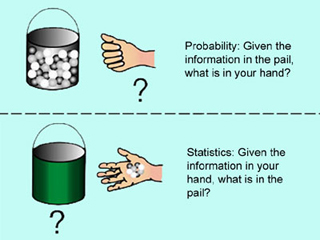14.30 | Spring 2006 | Undergraduate

# Introduction to Statistical Method in Economics

#### Course Description

This course is a self-contained introduction to statistics with economic applications. Elements of probability theory, sampling theory, statistical estimation, regression analysis, and hypothesis testing. It uses elementary econometrics and other applications of statistical tools to economic data. It also provides a …

## Course Info

##### Learning Resource Types
Exams with Solutions
Lecture Notes
Problem Sets with SolutionsDiagram showing the difference between statistics and probability. (Image by MIT OpenCourseWare. Based on Gilbert, Norma. Statistics. Philadelphia, PA: W. B. Saunders Co., 1976. ISBN: 072164127X.)